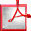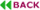The Concept of Unschedulability Core for Optimizing Priority Assignment in Real-Time Systems

Yecheng Zhaoa and Haibo Zengb
Department of Electrical and Computer Engineering, Virginia Tech, USA.
azyecheng@vt.edu
bhbzeng@vt.edu

ABSTRACT

In the design optimization of real-time systems, the schedulability analysis is used to define the feasibility region within which tasks meet their deadlines, so that optimization algorithms can find the best solution within the region. However, the complexity of current schedulability analysis techniques often makes it difficult to leverage existing optimization frameworks and scale to large designs. In this paper, we consider the design optimization problems for real-time systems scheduled with fixed priority, where task priority assignment is part of the decision variables. We propose the concept of unschedulability core, a compact representation of the schedulability conditions, and develop efficient algorithms for its calculation. We present a new optimization procedure based on lazy constraint paradigm that leverages such a concept. Experimental results on two case studies show that the new optimization procedure provides optimal solutions, but is a few magnitudes faster than other exact algorithms (Branch-and-Bound, Integer Linear Programming).Full Text (PDF)